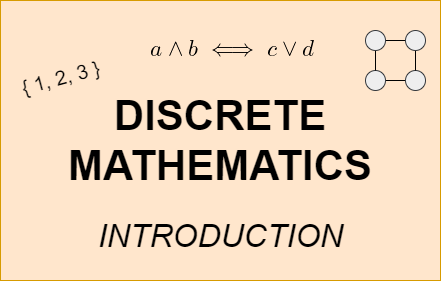# Mathematics - An Introduction to Discrete Mathematics## Introduction

Hey it's a me again @drifter1!

Today marks the beginning of a new Mathematics series on the branch of "Discrete Mathematics". Most students which study a branch of science which is related to math have some course on that exact topic. Some fundamental concepts are also covered during High School or even Middle School.

The branch of Discrete Mathematics deals with objects that have distinct / separate values, instead of continuous ranges of real numbers which are studied by branches such as Calculus. A broad range of unique subjects is covered by it, many of which are also commonly studied as completely separate branches by themselves! As such, today's post will be a small introduction to what Discrete Math is all about.

So, without further ado, let's get straight into it!

## Discrete Mathematics

Defining what exactly Discrete Mathematics is about, is difficult. The same can also be said for Mathematics though, as math is not simply the study of numbers, but also includes other concepts such as Functions or Geometry. Discrete Math is basically about studying mathematical structures that are distinct, separable and countable. For example, sets, graphs and logical statements are considered such structures.

It's also quite hard to specify which exact subjects are encapsulated by the branch of Discrete Mathematics. The subjects covered include fundamental concepts such as sets, relations, functions and sequences, mathematical statements, propositional logic, mathematical induction, recurrence relations, combinatorics, probability, statistics, graph theory, Boolean algebra and number theory.

Of course, not all of them will be covered thoroughly in this series, as some have been covered already more or less (like Boolean Algebra or Graph Theory) and some will get completely dedicated series later on (Probability, Statistics and Number Theory)...

## Why Discrete Math?

Even though the concepts covered by Discrete Math may seem unrelated to each other, there is quite a lot of cross-relation between them. But, the most important aspect of the branch, by far, is learning lots of different approaches for problem solving. It allows for creativity and is challenging. This is in contrast to Calculus, for example, where most problems have clear and perfectly fitting solutions for which specific mathematical equations and solving procedures must be used.

The kind of thinking that Discrete Math teaches is also very important for information and computer scientists to master. Using such a creative thinking approach its possible to solve most computational problems. That's also why Discrete Math finds a lot of applications in Computer Science in general, which is expected.

## Series Outline

As it was already mentioned, the branch covers a wide spectrum of unique topics. So, it's hard to say what exactly will be covered during the series and what not.

What can be said for sure though is that the series will start out from fundamental concepts, such as set theory and logic statements. Using set theory it's possible to explain most subjects covered by Discrete Math. This includes relations, functions, sequences etc. which will be explained in the context of discrete math. Logic statements are used for specifying problems in mathematical form. That's also when strategies for proving such statements, and basically solving mathematical problems will be covered, which for example includes mathematical induction.

Of course, there will also be posts on combinatorics, graphs, trees etc. which are all topics that are mostly discrete by nature. Lastly, a small introduction towards probability, statistics and number theory will also be made, covering the basics before the dedicated series come out.

And as always, either in the main post of each concept or in a separate post, there will be in-depth examples on all these concepts. So, don't worry if something seems too abstract when explained in a purely theoretical fashion!

## RESOURCES:

### References

Block diagrams and other visualizations were made using draw.io.

## Final words | Next up

And this is actually it for today's post!

In this blog I try to explain the most important topics in the simplest way possible. So, there will always be something that may be left out, mostly unintentionally. If anything is too difficult to understand, you can always reach out to me and the community at the comment section, and as a last resort even take a look at the references, which are always of top-notch quality!

Next time we will start covering the Fundamentals, with a post on Set Theory.

See ya!Keep on drifting!

Posted with STEMGeeks

0
0
0.000Great information! Thanks for sharing!

!1UP

0
0
0.000#### You have received a 1UP from @ivarbjorn!

The following @oneup-cartel family members will soon upvote your post:
`@stem-curator`
And they will bring !PIZZA 🍕